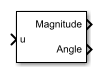# Fourier Analysis

Discrete or continuous time Fourier analysis

Since R2018b

•Libraries:
Simscape / Electrical / Control / General Control

## Description

The Fourier Analysis block performs a Fourier analysis on the input signal in either discrete or continuous time.

### Equations

A periodic function x(t) can be decomposed to an infinite sum of sine and cosine functions as

`$x\left(t\right)=\frac{{a}_{0}}{2}+\sum _{n=1}^{\infty }\left[{a}_{n}\mathrm{cos}\left(nt\right)+{b}_{n}\mathrm{sin}\left(nt\right)\right]$`

where:

• a0 is the DC component.

• an and bn are constant Fourier coefficients.

• n is the harmonic number.

The coefficients an and bn are defined as

`${\Omega }_{0}=\frac{2\pi }{{T}_{0}}$`

`${T}_{0}=\frac{1}{f}$`

where f is the fundamental frequency.

The magnitude and angle corresponding to the harmonic number are defined as:

`$|{X}_{n}|=\sqrt{{a}_{n}{}^{2}+{b}_{n}{}^{2}}$`

`${\theta }_{n}=-{\mathrm{tan}}^{-1}\left[\frac{{b}_{n}}{{a}_{n}}\right]$`

Note

If you use this block for continuous-time operations and set the Sample time (-1 for inherited) parameter to `0`, you should also specify the value of the Buffer size parameter to ensure it covers the moving time window.

## Ports

### Input

expand all

Input signal to be analyzed. The input can be a single signal or multiple multiplexed signals. Input signals can be AC currents or voltages in an electrical system.

Data Types: `single` | `double`

### Output

expand all

Signal magnitude corresponding to the harmonic number.

Data Types: `single` | `double`

Signal angle corresponding to the harmonic number.

Data Types: `single` | `double`

## Parameters

expand all

Fundamental frequency of the signal, in Hz. If you specify the fundamental frequency using a vector, it must match the input vector dimensions.

Specify signal harmonic numbers. Vector elements must be greater than or equal to 0.

Specify the initial magnitude of the signal. Vector elements must be greater than or equal to 0.

Initial phase angle of the signal, in rad.

Size of the buffer.

#### Dependencies

To enable this parameter, set Sample time (-1 for inherited) to `0`.

Time between consecutive block executions. During execution, the block produces outputs and, if appropriate, updates its internal state. For more information, see What Is Sample Time? and Specify Sample Time.

For inherited discrete-time operation, set this parameter to `-1`. For discrete-time operation, set this parameter to a positive integer. For continuous-time operation, set this parameter to `0`.

If this block is in a masked subsystem or a variant subsystem that supports switching between continuous operation and discrete operation, promote this parameter to ensure correct switching between the continuous and discrete implementations of the block. For more information, see Promote Block Parameters on a Mask.

## Version History

Introduced in R2018b